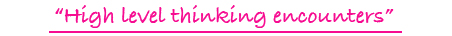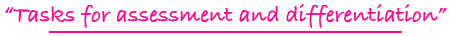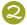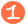•••Tiling Task Card Packets include 20 unique task cards on card stock, 3 backdrop cards, tracking sheets, suggestions and answer sheets.  These are contained in a printed manila envelope for convenient storing.  A set of number tiles 0 - 9 is  needed for each task card.

3 - 6

### LOGIC TILES DIGITAL

discovering a rule for computation and applying it; addition, subtraction, multiplication, division

Challenge Level:DIGITAL FORMAT\$10.00

3 - 6

### LOGIC TILES

discovering a rule for computation and applying it; addition, subtraction, multiplication, division

Challenge Level:PDF FORMAT
(You will receive both color and black and white files for printing.)\$10.00

5 - 8

### LOGIC TILES II

discovering the two-part rule, such as x2 - 3 and applying it; addition, subtraction, multiplication, division

Challenge Level:\$15.00

5 - 8

### LOGIC TILES II DIGITAL

discovering the two-part rule, such as x2 - 3 and applying it; addition, subtraction, multiplication, division

Challenge Level:DIGITAL FORMAT\$10.00

5 - 8

### LOGIC TILES II

discovering the two-part rule, such as x2 - 3 and applying it; addition, subtraction, multiplication, division

Challenge Level:PDF FORMAT
(You will receive both color and black and white files for printing.)\$10.00

5 - 8

### LOGIC TILES FOR ALGEBRA

forming algebraic expressions when x and y are given (symbol substitution)

Challenge Level:\$15.00

5 - 8

### LOGIC TILES FOR ALGEBRAIC THINKING

forming algebraic expressions after figuring the values of x and y

Challenge Level:\$15.00

5 - 8

### LOGIC TILES FOR ALGEBRAIC THINKING DIGITAL

forming algebraic expressions after figuring the values of x and y

Challenge Level:DIGITAL FORMAT\$10.00

5 - 8

### LOGIC TILES FOR ALGEBRAIC THINKING

forming algebraic expressions after figuring the values of x and y

Challenge Level:PDF FORMAT
(You will receive both color and black and white files for printing.)\$10.00

5 - 8

### LOGIC TILES: ALGEBRA DISCOVER Y

finding the value of y and completing the algebraic statements when given the value of x

Challenge Level:\$15.00

5 - 8

### LOGIC TILES: ALGEBRA X AND Y DIGITAL

finding the value of x and y and completing the algebraic statements by analyzing the given information

Challenge Level:DIGITAL FORMAT\$10.00

5 - 8

### LOGIC TILES: ALGEBRA X AND Y

finding the value of x and y and completing the algebraic statements by analyzing the given information

Challenge Level:PDF FORMAT
(You will receive both color and black and white files for printing.)\$10.00

K - 2

### LOOK AT PICTURE TILES DIGITAL

forming basic addition facts where pictures are used to help define addends and/or sums

Challenge Level:DIGITAL FORMAT\$10.00

K - 2

### LOOK AT PICTURE TILES

forming basic addition facts where pictures are used to help define addends and/or sums

Challenge Level:PDF FORMAT
(You will receive both color and black and white files for printing.)\$10.00

3 - 6

### LOOK! PATTERN BLOCK RELATIONSHIP TILES

finding values of geometric shapes when the value of one of the pattern blocks has been given; relationship thinking with the triangle, blue rhombus, trapezoid & hexagon

Challenge Level:\$15.00

3 - 5

### MEASURE UP TILES

estimating linear measurement with inches or centimeters; vocabulary of equal, more than, less than, between, double, and half of

Challenge Level:\$15.00

4 - 6

### METRIC MEASURE MAP TILES

applying a map scale where a centimeter is equal to a specific number of kilometers

Challenge Level:\$15.00

4 - 6

### METRIC MEASURE MAP TILES

applying a map scale where a centimeter is equal to a specific number of kilometers

Challenge Level:PDF FORMAT
(You will receive both color and black and white files for printing.)\$10.00

1 - 3

### MINUS TILES DIGITAL

forming basic subtraction facts with the help of pictorial images

Challenge Level:DIGITAL FORMAT\$10.00

1 - 3

### MINUS TILES

forming basic subtraction facts with the help of pictorial images

Challenge Level:PDF FORMAT
(You will receive both color and black and white files for printing.)\$10.00